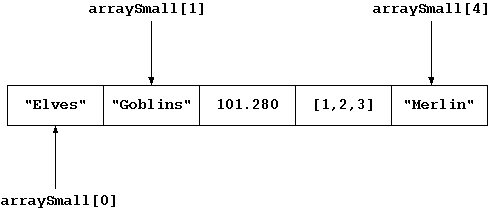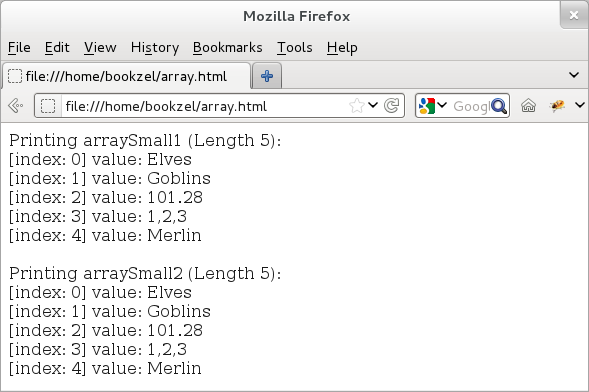## CodingBison

JavaScript arrays are an ordered list of values. The values are referred to as array elements. These values do not necessarily have to be of the same type. What this means is that a single array can contain elements of several types, including arrays as well!

JavaScript uses indexes to identify these elements. The first element has an index of 0, the second element has the next index of 1, and so on. Array indexes allow us to easily navigate the array. Even though JavaScript uses 64-bit storage for numbers, it still uses a 32-bit index for array indexes and thus, the largest possible index for an array element can be (2^32 - 1).

The following figure shows an example of a JavaScript array ("arraySmall") with 5 elements.Figure: A JavaScript Array with 5 elements

The first step to using an array is to define it. For this task, JavaScript offers two methods. In the first method, we can define an array ("arraySmall") using square brackets as "var arraySmall = []"; we do not have to specify the size of the array. In the second method, we can take advantage of the fact that JavaScript arrays are actually objects. Being objects, JavaScript Arrays come with a built-in constructor: "Array()" and we can use this constructor to define the array: "var arraySmall= new Array()".

When we use the typeof operator of an array, then it returns "object" instead of, let us say "array". This is okay since arrays are in fact objects. However, JavaScript supports many types of objects and if we want to confirm that an object is indeed an array type object, then we can use the isArray() method provided by the Array constructor: isArray(). This method returns true (boolean) if the object passed is an array, else, it returns false. Thus "Array.isArray(arraySmall)" would return true for the "arraySmall" array.

Since JavaScript arrays are objects, when we attempt to access an element as "arraySmall", internally, JavaScript converts the index into a string as "arraySmall["1"]" and then uses the string ("1") as the name of the object property. The value stored at that index is actually the value of this property.

JavaScript arrays are flexible when it comes to adding and deleting elements -- we can add and delete elements to arrays dynamically.

To add an element, we can simply identify the element using a new index and assign a value to it; e.g. we can add a new (sixth) element to arraySmall of above figure as: "arraySmall = 680"; an index of 5 means that the new element is in fact the sixth element.

Sometimes, we may find it more compact to use a single step to not only define an array but to also add some initial values to it. To do this, both of the above array-definition methods accept initial values. For the first case, we can use "var arraySmall = ["Elves", "Goblins", 101.280, [1,2,3], "Merlin"] ". For the second case, we can use "var arraySmall = new Array("Elves", "Goblins", 101.280, [1,2,3], "Merlin")".

However, the Array constructor has one trick up its sleeve -- if the argument to Array() is a single number, then JavaScript interprets it as the length of the array. Hence, "var arraySmall = new Array(5)" would define an array with length 5 and not an array with an initial value of 5! However, with "var arraySmall = new Array("5")", JavaScript would not define an array with 5 elements since "5" is not a number.

To delete an element, we can use the delete operator; e.g. we can delete the first element of the arraySmall as: "delete arraySmall". In fact, JavaScript provides a host of built-in methods for inserting and deleting elements as well. We will talk more about these methods later in this chapter.

When we delete an array element using the "delete" operator, then JavaScript does not actually delete that element; instead, it simply replaces the value stored at that index with "undefined" value. Because of this, the array length remains unchanged when we use the delete operator; arrays come with a default "length" property that stores array length.

This unique behavior of delete means that the largest index of an array may not necessarily be equal to the (array length -1) since it is possible that one can delete the largest index. Thus, with JavaScript arrays, all we can say is that the array length must be numerically greater than or equal to (anyIndex + 1), where anyIndex can be index of any of its elements.

In fact, if we set an array length to a lower value than the current one, then JavaScript automatically deletes all the elements that lie beyond the new length. In other words, if an array has 10 elements and if we set its length to 5, then JavaScript would delete the last 5 elements of the array.

Before we go any further, let us use a simple example to demonstrate array definition. This example (provided below) uses both of these methods to define arraySmall. Further, this example implements a "printArray()" function to print array elements.

``` <!doctype html>
<html>
<script type="text/javascript">

// A simple function to print array elements
function printArray (passedArray) {
for (var i=0; i < passedArray.length; i++) {
document.write("[index: " + i + "] value: " + passedArray[i] + "<br>");
}
}

// Define arraySmall1 using the "[]" method
var arraySmall1 = ["Elves", "Goblins", 101.280, [1,2,3],  "Merlin"];
document.write("Printing arraySmall1 (Length " + arraySmall1.length + "):<br>");
printArray(arraySmall1);

// Define arraySmall2 using the "new Array()" method
var arraySmall2 = new Array("Elves", "Goblins", 101.280, [1,2,3],  "Merlin");
document.write("<br>Printing arraySmall2 (Length " + arraySmall2.length + "):<br>");
printArray(arraySmall2);

</script>
</html>
```

The output (provided below) prints array elements identically for both arrays. Note to self, this being the first example should be provided as an image and not as a simple text output.Figure: JavaScript Arrays

### Iterating Array Elements using a for/in Loop

The above example uses a "for" loop to iterate through array elements. Besides a for loop, we can also use JavaScript's (custom) "for/in" loop to iterate Array elements. The for/in loop enumerates all the (enumerable) properties of an object and since arrays are objects, we can conveniently use this loop to iterate through Array properties, which are in fact array indexes.

Nevertheless, using for/in loops comes with two cautionary notes. First, when it lists object properties (and in this case array indexes), it may not list them in the exact order as they were defined. If ordering is important, we should stick with using a for loop. Second, with arrays, it may skip those records that have been deleted.

Let us understand the behavior of for/in loop using an example. In this example (provided below), we reuse "arraySmall" array from the earlier example. We begin by deleting the first two elements of this array and then, print array elements using both for and for/in loops. Since with delete operation, JavaScript merely replaces the value of the deleted element with "undefined" value, the for loop confirms this behavior and shows records with "undefined" values. However, the for/in skips those records with "undefined" value.

``` <!doctype html>
<html>
<div id="idDiv"></div>

<script type="text/javascript">
// Get a handle of the div element.
var elem = document.getElementById("idDiv");

// Define an arraySmall with some initial values
var arraySmall = ["Elves", "Goblins", 101.280, [1,2,3],  "Merlin"];

// And, then delete some of the array elements
delete arraySmall;
delete arraySmall;

// Print the length
elem.innerHTML += "After deletion, the Length is: " + arraySmall.length + "<br>";

// Print using a for loop
elem.innerHTML += "<br>Printing using a for loop: <br>";
for (var i=0; i < arraySmall.length; i++) {
elem.innerHTML += "[index: " + i + "] value: " + arraySmall[i] + "<br>";
}

// Print using a for/in loop
elem.innerHTML += "<br>Printing using a for/in loop: <br>";
for (var j in arraySmall) {
elem.innerHTML += "[index: " + j + "] value: " + arraySmall[j] + " <br>";
}
</script>
</html>
```

We provide the output below. As explained earlier, the length of the array remains unchanged even after deleting its elements.

``` After deletion, the Length is: 5

Printing using a for loop:
[index: 0] value: undefined
[index: 1] value: undefined
[index: 2] value: 101.28
[index: 3] value: 1,2,3
[index: 4] value: Merlin

Printing using a for/in loop:
[index: 2] value: 101.28
[index: 3] value: 1,2,3
[index: 4] value: Merlin
```